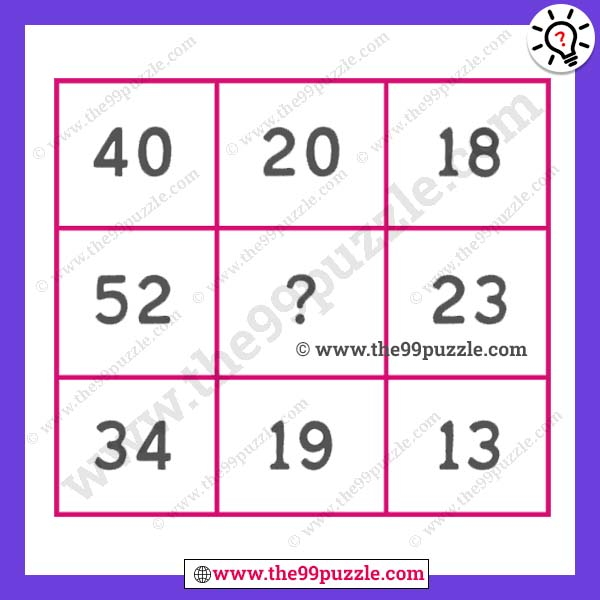# Simple mathematical puzzle tricks with answer – Puzz278

Only genius people can solve these simple mathematical puzzle tricks. If you consider yourself a genius then you must answer this question. Here you will find new math puzzles every day. Each puzzle will improve your math skills. These types of maths come in every competitive exam. If you practice these math puzzles every day then it will be easier to crack any of your exams and you will be able to solve them in less time. There is a trick to this math puzzle. First, you have to find the tricks of this mathematics.###### Explanation:

1st Row = (40-18) = 22-2 = 20

2nd Row = (52-23) = 29-2 = 27

3rd Row = (34-13) = 21-2 = 19